Ex 13.3

Chapter 13 Class 10 Surface Areas and Volumes
Serial order wise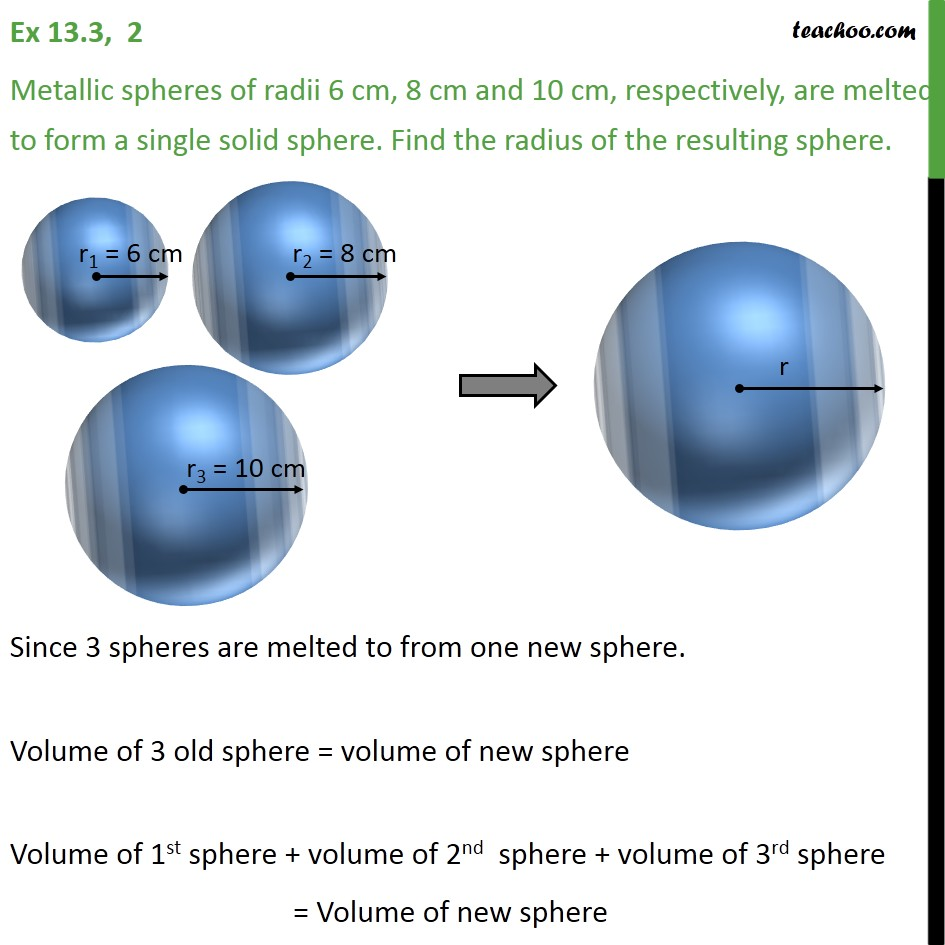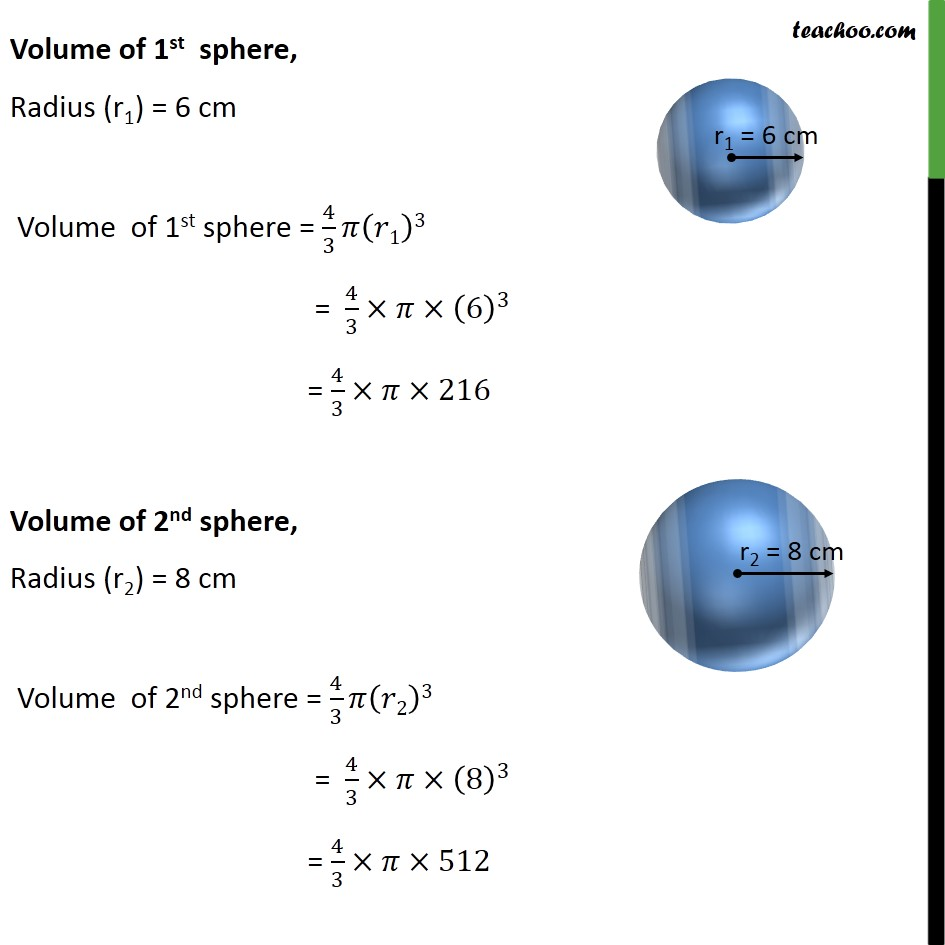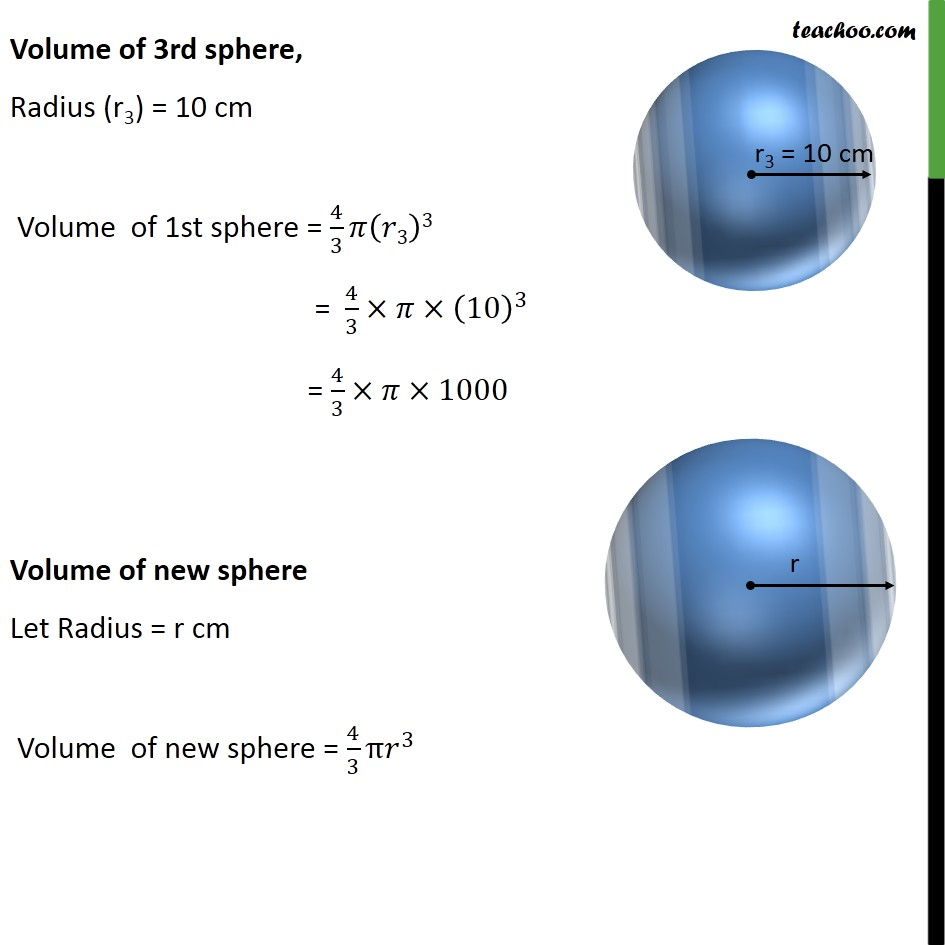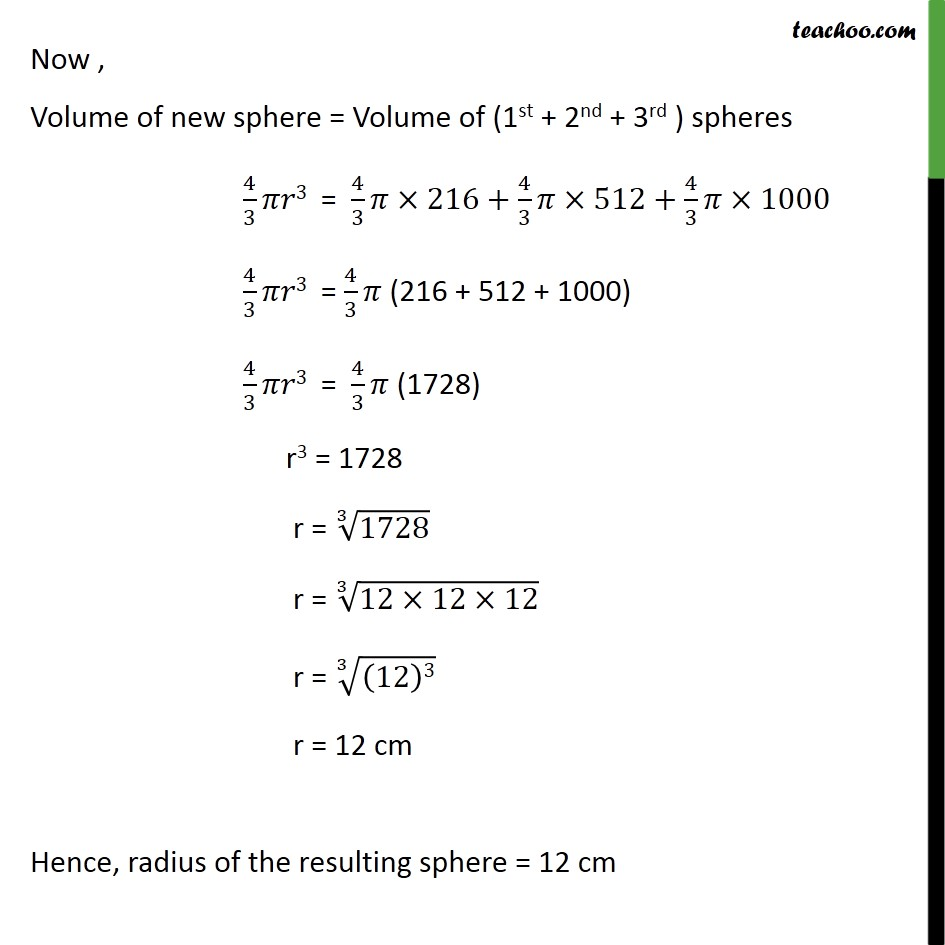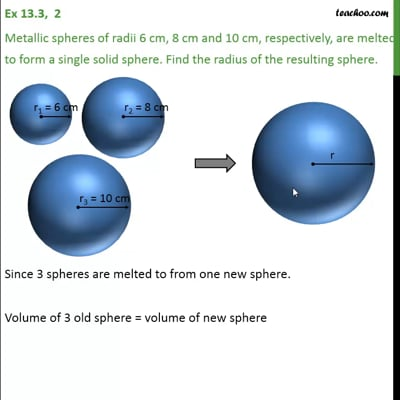This video is only available for Teachoo black users

Solve all your doubts with Teachoo Black (new monthly pack available now!)

### Transcript

Ex 13.3, 2 Metallic spheres of radii 6 cm, 8 cm and 10 cm, respectively, are melted to form a single solid sphere. Find the radius of the resulting sphere. Since 3 spheres are melted to from one new sphere. Volume of 3 old sphere = volume of new sphere Volume of 1st sphere + volume of 2nd sphere + volume of 3rd sphere = Volume of new sphere Volume of 1st sphere, Radius (r1) = 6 cm Volume of 1st sphere = 4/3 𝜋(𝑟1)3 = 4/3×𝜋×(6)^3 = 4/3×𝜋×216 Volume of 2nd sphere, Radius (r2) = 8 cm Volume of 2nd sphere = 4/3 𝜋(𝑟2)3 = 4/3×𝜋×(8)^3 = 4/3×𝜋×512 Volume of 3rd sphere, Radius (r3) = 10 cm Volume of 1st sphere = 4/3 𝜋(𝑟3)3 = 4/3×𝜋×(10)^3 = 4/3×𝜋×1000 Volume of new sphere Let Radius = r cm Volume of new sphere = 4/3 π𝑟^3 Now , Volume of new sphere = Volume of (1st + 2nd + 3rd ) spheres 4/3 𝜋𝑟3 = 4/3 𝜋×216+4/3 𝜋×512+4/3 𝜋×1000 4/3 𝜋𝑟3 = 4/3 𝜋 (216 + 512 + 1000) 4/3 𝜋𝑟3 = 4/3 𝜋 (1728) r3 = 1728 r = ∛1728 r = ∛(12×12×12) r = ∛((12)3) r = 12 cm Hence, radius of the resulting sphere = 12 cm